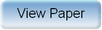Ninth International Geostatistics Congress, Oslo, Norway
June 11 – 15, 2012Session: Plenary 6 Abstract No.: O-050 Title: On the construction of discrete distributions from training images Author(s): H. Tjelmeland, Norwegian University of Science and Technology (NO)H. Toftaker, Norwegian University of Science and Technology (NO) Abstract: Bayesian modelling requires a prior distribution and a likelihood model to be specified. The likelihood is typically based on the physical understanding of the observation process. In reservoir characterisation is has become common practice to adopt an empirical Bayes approach by estimating the prior from one or more training images. In the case of discrete prior models multi-point statistics are frequently used. In multi-point statistics the node values are simulated sequentially in a random order and conditional distributions for one node value given the previous are estimated from the training image(s). The number of model parameters to estimate is typically huge and thereby the estimation uncertainty may become a problem. The estimated conditional distributions define a (higher-order) Markov chain. The estimated Markov chain will not be stationary unless restricted to be so by the estimation process. The random order of node simulation used in multi-point statistics is hiding, but not removing, the effect of the non-stationarity. In this paper we focus on binary training images and discuss how to cope with the two problems discussed above. As in multi-point statistics we simulate the node values sequentially, but in a fixed order. To reduce the number of model parameters that need to be estimated from the training image(s) we parameterise the conditional distributions by a small number of parameters. To get a sufficiently flexible model we define a hyper-prior for how many and what model parameters to include. Thus, we get a hierarchical Bayesian model, which we fit to the given training image(s) by a reversible jump algorithm. Thereby we are also able to represent the uncertainty of the estimated prior. As a part of the hyper-prior we restrict the conditional distributions to be so that the induced Markov chain is stationary. One should also note that the fixed simulation order we are using for the node values imply that we easily can evaluate the prior density of a given realisation, which in turn means that the Metropolis--Hastings algorithm can be used to generate conditional simulations.Produced by X-CD Technologies Inc.